# Olympiad Test Level 1: Numbers - 1

## 10 Questions MCQ Test Math Olympiad for Class 2 | Olympiad Test Level 1: Numbers - 1

Description
Attempt Olympiad Test Level 1: Numbers - 1 | 10 questions in 20 minutes | Mock test for Class 2 preparation | Free important questions MCQ to study Math Olympiad for Class 2 for Class 2 Exam | Download free PDF with solutions
QUESTION: 1

Solution:

456 < 465 < 546

QUESTION: 2

### Choose the incorrect one:

Solution:

552 is not equal to 550.

QUESTION: 3

### Find odd one out

Solution:
QUESTION: 4

How many tens are there in 43?

Solution:

It has 4 tens.

QUESTION: 5

In 43 the digit at the ones place is:

Solution:
QUESTION: 6

Find the number from the following which has 5 at tens place and 4 at unit place ?

Solution: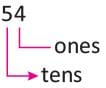QUESTION: 7

Find the number represented by the following figure: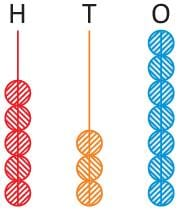Solution:
QUESTION: 8

Which one of the following numbers has 5 tens 6 ones ?

Solution: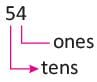QUESTION: 9

Which is incorrectly matched?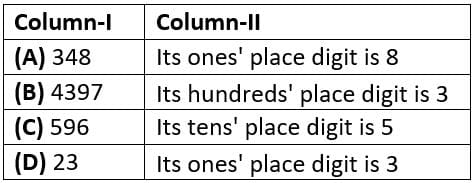Solution:

As its tens place digit is 9.

QUESTION: 10

The digit at the hundreds place in the number 523 is ___________ .

Solution: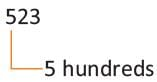Use Code STAYHOME200 and get INR 200 additional OFF Use Coupon Code